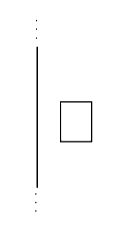# Current Loop Rotate Question

• syhpui2

## Homework Statement

Consider a long wire running in the vertical direction with a rectangular loop of wire beside it as shown. Now suppose that a constant current, I, flows upward along the long wire, and the same magnitude current, I, flows clockwise in the loop.

As viewed from the top of the page, in which direction does the loop rotate?

(a) clockwise
(b) counterclockwise
(c) The loop does not rotate.http://i.imgur.com/n6Vbw.png

F=BIXL

## The Attempt at a Solution

I tried use RHR with loop. However, I get answer B because force points to right...

With the current running toward the top of the diagram, which way do its magnetic field lines intersect the sides of the rectangular loop? We know the magnetic field is stronger on the side of the loop closer to the wire, BUT which way does the magnetic force act on the current in each of the four sides of the loop? The important question is: do these forces on the sides of the loop act in a way that will turn the loop out of the plane it is presently lying in?

With the current running toward the top of the diagram, which way do its magnetic field lines intersect the sides of the rectangular loop? We know the magnetic field is stronger on the side of the loop closer to the wire, BUT which way does the magnetic force act on the current in each of the four sides of the loop? The important question is: do these forces on the sides of the loop act in a way that will turn the loop out of the plane it is presently lying in?

do these forces on the sides of the loop act in a way that will turn the loop out of the plane it is presently lying in?

i thought the force on left edge is stronger because it is closer so it would rotate clockwise?

i thought the force on left edge is stronger because it is closer so it would rotate clockwise?

To decide whether any rotation will occur, you need to figure out the direction of the forces acting on all four sides. The strength of the forces alone don't decide that question.

To decide whether any rotation will occur, you need to figure out the direction of the forces acting on all four sides. The strength of the forces alone don't decide that question.

Okay. That makes sense.
Thanks